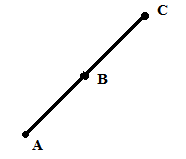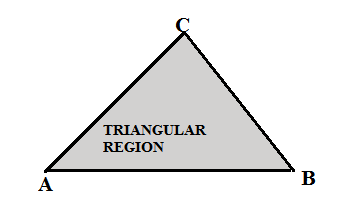RD Sharma 2013 Solutions for Class 6 Math Chapter 12 Triangles are provided here with simple step-by-step explanations. These solutions for Triangles are extremely popular among class 6 students for Math Triangles Solutions come handy for quickly completing your homework and preparing for exams. All questions and answers from the RD Sharma 2013 Book of class 6 Math Chapter 12 are provided here for you for free. You will also love the ad-free experience on Meritnation’s RD Sharma 2013 Solutions. All RD Sharma 2013 Solutions for class 6 Math are prepared by experts and are 100% accurate.

#### Question 1:

Take three non-collinear points A, B and C on a page of your notebook. Join AB, BC and CA.
What figure do you get? Name the triangle. Also, name

(i) the side opposite to ∠B
(ii) the angle opposite to side AB
(iii) the vertex opposite to side BC
(iv) the side opposite to vertex B.

#### Answer:

Let us consider three non-collinear points A, B and C and join them.
After joining these points, we get a 'Triangle', as it consists of three sides. The name of the triangle we get is ▲ABC.(i) The side opposite ∠B is AC.
(ii) The angle opposite side AB is ∠C.
(iii) The vertex opposite side BC is A.
(iv) The side opposite vertex B is AC.

#### Question 2:

Take three collinear points A, B and C on a page of your note book. Join AB, BC and CA. Is the figure a triangle ? If not, why?

#### Answer:

Let us consider three collinear points A, B and C and join AB, BC and CA.The figure we get is not a triangle because it is a straight line consisting of only one side. It is also not a closed figure, whereas a triangle is defined as a closed figure consisting of three sides.

#### Question 3:

Distinguish between a triangle and its triangular region.

#### Answer:

A triangle is defined as a closed polygon consisting of three sides, whereas a triangular region is the region that lies inside the triangle. In the adjoining figure, the shaded region shows the triangular region.#### Question 4:

In Fig. 12.11, D is a point on side BC of a ∆ABC. AD is joined. Name all the triangles that can observe in the figure. How many are they?#### Answer:

The figure consists of triangles ∆ADC, ∆ADB and ∆ABC. Therefore, three triangles are present in the figure.#### Question 5:

In Fig. 12.12, A, B, C and D are four points, and no three points are collinear. AC and BC intersect at O. There are eight triangles that you can observe. Name all the triangles.#### Answer:

The following figure consists of triangles, namely ∆ODC, ∆ODA, ∆OBC, ∆OAB, ∆ADB, ∆ACB, ∆DAC and ∆DBC. Hence, there are a total of eight triangles.#### Question 6:

What is the difference between a triangle and triangular region?

#### Answer:

A triangle is defined as a closed polygon consisting of three sides, whereas a triangular region is the region that lies inside the three sides of the triangle.

In the adjoining figure, the shaded region shows the triangular region.#### Question 7:

Explain the following terms:

(i) Triangle
(ii) Parts or elements of a triangle
(iii) Scalene triangle
(iv) Isosceles triangle
(v) Equilateral triangle
(vi) Acute triangle
(vii) Right triangle
(viii) Obtuse triangle
(ix) Interior of a triangle
(x) Exterior of a triangle.

#### Answer:

(i) Triangle - a triangle is a closed polygon that consists of three straight lines as its sides.
(ii) Parts or elements of a triangle - a triangle consists of three sides, three angles and three vertices.
(iii) Scalene triangle - a triangle, in which the length of all the sides are different.
(iv) Isosceles triangle - a triangle, in which the length of two sides are equal.
(v) Equilateral triangle - a triangle, in which the length of all the sides are equal.
(vi) Acute triangle - a triangle, in which all the angles measure less than 90o.
(vii) Right triangle - a triangle, which has an angle that measures 90o.
(viii) Obtuse triangle - a triangle, in which one of the angles measure more than 90o.
(ix) Interior of a triangle - the region lying inside the boundaries or sides of a triangle.
(x) Exterior of a triangle - the region lying outside the boundaries or sides of a triangle.

#### Question 8:

In Fig. 12.13, the length (in cm) of each side has been indicated along the side. State for each triangle wheather it is scalene, isosceles or equilateral:#### Answer:

(i) This is a scalene triangle, as all the sides have different length.
(ii) This is an isosceles triangle, as two sides are equal in length i.e. 5.6 cm.
(iii) This is an equilateral triangle, as all the sides are equal in length i.e. 5 cm.
(iv) This is a scalene triangle, as all the sides have different length.
(v) This is an isosceles triangle, as two sides are equal in length i.e. 6.2 cm.

#### Question 9:

In Fig. 12.14, there are five triangles. The measures of some of their angles have been indicated. State for each triangle whether it is acute, right or obtuse.#### Answer:

(i) This is a right angled triangle, as it contains a 90o angle.
(ii) This is an obtuse angled triangle, as one of the angle(120o) measures more than 90o and less than 180o.
(iii) This is an acute angled triangle, as all the angles are less than 90o.
(iv) This is a right angled triangle, as it contains a 90o angle.
(v) This is an obtuse angled triangle, as one of the angle (110o) measures more than 90oand less than 180o.

#### Question 10:

Fill in the blanks with the correct word/symbol to make it a true statement:

(i) A triangle has .......sides.
(ii) A triangle has .......vertices.
(iii) A triangle has .......angles.
(iv) A triangle has .......parts.
(v) A triangle whose no two sides are equal is known as .....
(vi) A triangle whose two sides are equal is known as ....
(vii) A triangle whose all the sides are equal is known as......
(viii) A triangle whose one angle is a right angle is known as......
(ix) A triangle whose all the angles are of measure less than 90° is known as....
(x) A triangle whose one angle is more than 90° is known as ......

#### Answer:

(i) A triangle has three sides.
(ii) A triangle has three vertices.
(iii) A triangle has three angles.
(iv) A triangle has six parts.
(Angles and sides are part of a triangle. So, three angles and three sides make six parts).
(v) A triangle whose no two sides are equal is known as Scalene triangle.
(A triangle whose length of all sides are different is called Scalene triangle).
(vi) A triangle whose two sides are equal is known as Isosceles triangle.
(A triangle whose length of two sides are equal is called an Isosceles triangle).
(vii) A triangle whose all the sides are equal is known as Equilateral triangle.
(A triangle whose length of all sides are equal is called an Equilateral triangle).
(viii) A triangle whose one angle is a right angle is known as Right angled triangle.
(A triangle whose one angle is 90° is called a Right angled triangle).
(ix) A triangle whose all angles measure less than 90° is known as an Acute triangle.
(A triangle whose all angles are less than 90° is called an Acute triangle).
(x) A triangle whose one angle is more than 90° is known as an Obtuse triangle.
(A triangle whose one angle is more than 90° is called an Obtuse triangle).

#### Question 11:

In each of the following, state if the statement is true (T) or false (F):

(i) A triangle has three sides.
(ii) a triangle may have four vertices.
(iii) Any three line-segments make up a triangle.
(iv) The interior of a triangle includes its vertices.
(v) The triangular region includes the vertices of the corresponding triangle.
(vi) The vertices of a triangle are three collinear points.
(vii) An equilateral triangle is isosceles also.
(viii) Every right triangle is scalene.
(ix) Each acute triangle is equilateral.
(x) No isosceles triangle is obtuse.

#### Answer:

(i) T
(ii) F; a triangle consists of three vertices only.
(iii) F; three line segments joined by three non-collinear points can only form a triangle.
(iv) F; it lies on the triangle.
(v) T
(vi) F; the vertices of a triangle are three non-collinear points.
(vii) T
(viii) F; it can also be an isosceles triangle.
(ix) F; it can also be a scalene or an isosceles triangle.
(x) F; it can be an obtuse triangle.

#### Question 1:

Total number of parts of a triangle is

(a) 3
(b) 6
(c) 9
(d) 1

#### Answer:

(b) 6
Three sides and three angles.

#### Question 2:

A perpendicular drawn from a vertex to the opposite side of a triangle is known as

(a) an altitude
(b) a median
(c) an angle bisector
(d) a bisector

#### Answer:

(a) An altitude
An altitude is defined as the perpendicular drawn from a vertex to the opposite side of a triangle.

#### Question 3:

A triangle

(a) may not have an altitude
(b) can have at most 3 altitudes
(c) has three altitudes
(d) has only one altitude

#### Answer:

(c) has three altitudes

#### Question 4:

Line segments joining the vertices to the mid-points of the opposite sides of a triangle are known as

(a) medians
(b) altitudes
(c) heights
(d) angle bisectors

#### Answer:

(a) medians
A median is defined as the line segment joining the vertex to the mid-point of the opposite side of a triangle.

#### Question 5:

A triangle whose no two sides are equal is known as

(a) an acute triangle
(b) a scalene triangle
(c) an isosceles triangle
(d) an equilateral triangle

#### Answer:

(b) A Scalene triangle
A Scalene triangle is defined as the triangle in which no sides are equal.

#### Question 6:

A triangle whose two sides are equal is known as

(a) acute triangle
(b) an isosceles triangle
(c) a scalene triangle
(d) an isosceles triangle

#### Answer:

(b) An isosceles triangle
An isosceles triangle is a triangle that has two equal sides.

#### Question 7:

A triangle whose all sides are equal is called

(a) an equilateral triangle
(b) an acute triangle
(c) a right triangle
(d) an isosceles triangle

#### Answer:

(a) An equilateral triangle
An Equilateral triangle is defined as a triangle whose all sides are equal.

#### Question 8:

The sum of the length of sides of a triangle is known as its

(a) area
(b) height
(c) perimeter
(d) region

#### Answer:

(c) Perimeter
Perimeter is defined as the sum of the length of all the sides of a triangle.

#### Question 9:

A triangle having all sides of different length is known as

(a) scalene triangle
(b) isosceles triangle
(c) an equilateral triangle
(d) an acute triangle

#### Answer:

(a)  Scalene triangle.
A Scalene triangle is defined as a triangle having all sides of different length.

#### Question 10:

A triangle whose one angle is more than 90° is called

(a) a scalene triangle
(b) a right triangle
(c) an acute triangle
(d) an obtuse triangle

#### Answer:

(d) An obtuse triangle.
An obtuse triangle is a triangle whose one angle is more than 90°.

View NCERT Solutions for all chapters of Class 6